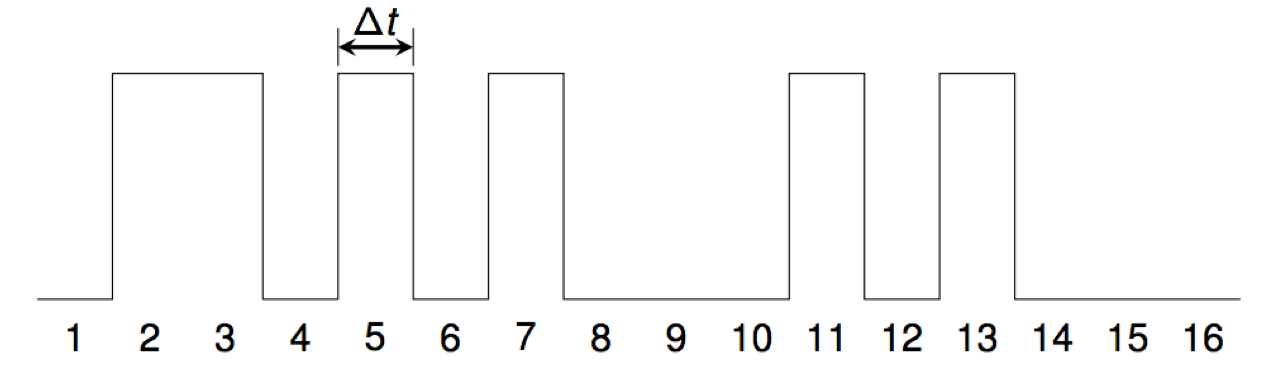# Number Theory and Noise

## Joo Young "Jon" Kim, Erik Huang, Pooja Ramanathan

This project investigates the representations of sets of positive integers (sequences) as sound.

A digital audio waveform is created from a given set A of positive integers by setting sample number i to a non-zero constant c for all i in the set. All other samples are set to zero.

For example, the waveform for the primes starts like this:We use the standard CD-audio sampling rate of 44100 samples per second, so Δt = 1/44100= 0.0000226757... seconds.

For many sets, the result is what most people would describe as noise.

## OEIS sequences

A000069 Odious numbers: numbers with an odd number of 1's in their binary expansion. download Erik Huang Aut2018
A000567 Octagonal numbers: n*(3*n-2). Also called star numbers. download Erik Huang Aut2018
A002061 Central polygonal numbers: a(n) = n^2 - n + 1. download Erik Huang Aut2018
A002808 The composite numbers: numbers n of the form x*y for x > 1 and y > 1. download Erik Huang Aut2018
A006753 Smith numbers: composite numbers n such that sum of digits of n = sum of digits of prime factors of n (counted with multiplicity). download Erik Huang Aut2018
A006995 Binary palindromes: numbers whose binary expansion is palindromic. download Erik Huang Aut2018
A007602 Numbers that are divisible by the product of their digits. download Erik Huang Aut2018
A007770 Happy numbers: numbers whose trajectory under iteration of sum of squares of digits map includes 1. download Erik Huang Aut2018
A014190 Palindromes in base 3 (written in base 10). download Erik Huang Aut2018
A014192 Palindromes in base 4 (written in base 10). download Erik Huang Aut2018
A014486 List of totally balanced sequences of 2n binary digits written in base 10. Binary expansion of each term contains n 0's and n 1's and reading from left to right (the most significant to the least significant bit), the number of 0's never exceeds the number of 1's. download Erik Huang Aut2018
A018252 The nonprime numbers (1 together with the composite numbers). download Erik Huang Aut2018
A029803 Numbers that are palindromic in base 8. download Erik Huang Aut2018
A052223 Numbers whose sum of digits is 9. download Erik Huang Aut2018
A072543 Numbers whose largest decimal digit is also the initial digit (to 107) download Erik Huang Aut2018
A153880 Shift factorial base representation left by one digit (to 4 million) download Erik Huang Aut2018
A166459 Numbers whose sum of digits is 19. download Erik Huang Aut2018
A235151 Numbers whose sum of digits is 12. download Erik Huang Aut2018
A235229 Numbers whose sum of digits is 20. download Erik Huang Aut2018
A243615 Numbers n whose digital sum equals the number of binary digits in its binary expansion. download Erik Huang Aut2018
A243617 Numbers n whose sum of digits equals the number of bits in its binary expansion. No zeros allowed in the digital expansion. download Erik Huang Aut2018
A003622 The Wythoff compound sequence AA: [n*phi^2] - 1, where phi = (1+sqrt(5))/2. download Pooja Ramathan Aut2018 beatty
A005728 Number of fractions in Farey series of order n. download Pooja Ramathan Aut2018